# Lathyrus vernus function-based age-by-stage MPMs

This document was built in Markdown in R 4.1.1 and compiled on 06 September 2021. It covers package lefko3 version 3.8.0. Please note that this vignette was written with space considerations in mind. To reduce output size, we have prevented some statements from running if they produce long stretches of output. Examples include most summary() calls. In these cases, we include hashtagged versions of these calls, and our text assumes that the user runs these statements without hashtags.

## CASE STUDIES OF SWEDISH Lathyrus vernus POPULATION

In this vignette, we use the lathyrus dataset to illustrate the estimation of age-by-stage function-based MPMs. These are inherently ahistorical MPMs, and so we do not include historical analyses in this vignette. Please see the other vignettes included in package lefko3, as well as further vignettes posted online on the projects page of the Shefferson lab website, for further demonstrations of raw MPMs, function-based MPMs, IPMs, and age-by-stage MPMs.

### ORGANISM AND POPULATION

Lathyrus vernus (family Fabaceae) is a long-lived forest herb, native to Europe and large parts of northern Asia. Individuals increase slowly in size and usually flower only after 10-15 years of vegetative growth. Flowering individuals have an average conditional lifespan of 44.3 years (Ehrlén and Lehtilä 2002). L. vernus lacks organs for vegetative spread and individuals are well delimited (Ehrlén 2002). One or several erect shoots of up to 40 cm height emerge from a subterranean rhizome in March-April. Flowering occurs about four weeks after shoot emergence. Shoot growth is determinate, and the number of flowers is determined in the previous year (Ehrln and Van Groenendael 2001). Individuals may not produce aboveground structures every year, but can remain dormant in one or more seasons. L. vernus is self-compatible but requires visits from bumble-bees to produce seeds. Individuals produce few, large seeds and establishment from seeds is relatively frequent (Ehrlén and Eriksson 1996). The pre-dispersal seed predator Bruchus atomarius often consumes a large fraction of developing seeds, and roe deer (Capreolus capreolus) sometimes consume the shoots (Ehrlén and Münzbergová 2009).

Data for this study were collected from six permanent plots in a population of L. vernus located in a deciduous forest in the Tullgarn area, SE Sweden (58.9496 N, 17.6097 E), during 1988–1991 (Ehrlén 1995). The six plots were similar with regard to soil type, elevation, slope, and canopy cover. Within each plot, all individuals were marked with numbered tags that remained over the study period, and their locations were carefully mapped. New individuals were included in the study in each year. Individuals were recorded at least three times every growth season. At the time of shoot emergence, we recorded whether individuals were alive and produced above-ground shoots, and if shoots had been grazed. During flowering, we recorded flower number and the height and diameter of all shoots. At fruit maturation, we counted the number of intact and damaged seeds. To derive a measure of above-ground size for each individual, we calculated the volume of each shoot as $$\pi × (\frac{1}{2} diameter)^2 × height$$, and summed the volumes of all shoots. This measure is closely correlated with the dry mass of aboveground tissues ($$R^2 = 0.924$$, $$P < 0.001$$, $$n = 50$$, log-transformed values; Ehrlén 1995). Size of individuals that had been grazed was estimated based on measures of shoot diameter in grazed shoots, and the relationship between shoot diameter and shoot height in non-grazed individuals. Only individuals with an aboveground volume of more than 230 mm3 flowered and produced fruits during this study. Individuals that lacked aboveground structures in one season but reappeared in the following year were considered dormant. Individuals that lacked aboveground structures in two subsequent seasons were considered dead from the year in which they first lacked aboveground structures. Probabilities of seeds surviving to the next year, and of being present as seedlings or seeds in the soil seed bank, were derived from separate yearly sowing experiments in separate plots adjacent to each subplot (Ehrlén and Eriksson 1996).

### OVERALL GOALS AND INITIAL CONSIDERATIONS

Our goal in this exercise will be to produce ahistorical, age-by-stage function-based matrices using the Lathyrus dataset. We will use a different approach to size classification than in previous vignettes, using the natural log of size instead of the normal size shown in the dataset. Our reasoning has to do with the fact that volume is used as the size metric here, and so should have an allometric relationship to some vital rates (note that all size metrics have allometric relationships, but this is clearer when size is based on something more strongly related to mass, as is volume). We will also create both reproductive and non-reproductive stages of the same size classes.

The dataset that we have provided is organized in horizontal format, meaning that each row holds all of the data for a single, unique individual, and columns correspond to individual condition in particular monitoring occasions (which we refer to as years here, since there was one main census in each year). The original spreadsheet file used to keep the dataset has a repeating pattern to these columns, with each year having a similarly arranged group of variables. Package lefko3 includes functions to handle data in horizontal format, as well as vertically formatted data (i.e. data for individuals is broken up across rows, where each row is a unique combination of individual and year in occasion t).

First, let’s clear memory and load the dataset.

rm(list=ls(all=TRUE))
library(lefko3)
data(lathyrus)
#summary(lathyrus)

This dataset includes information on 1,119 individuals, so there are 1,119 rows with data (not counting the header). There are 38 columns. The first two columns are variables identifying each individual (SUBPLOT refers to the patch, and GENET refers to individual identity), with each individual’s data entirely restricted to one row. This is followed by four sets of nine columns, each named VolumeXX, lnVolXX, FCODEXX, FlowXX, IntactseedXX, Dead19XX, DormantXX, Missing19XX, and SeedlingXX, where XX corresponds to the year of observation and with years organized consecutively. Thus, columns 3-11 refer to year 1988, columns 12-20 refer to year 1989, etc. This strictly repeated pattern allows us to manipulate the original dataset quickly and efficiently via lefko3. We should know the total number of years of data in the dataset, which is 4 years here (includes all years from and including 1988 to 1991). Ideally, we should also have arranged the columns in the same order for each year, with years in consecutive order with no extra columns between them. Note that this order is not required, but it makes life easier because following a strictly repeating pattern allows us to skip inputting the names or numbers of each column of data directly later during demographic data formatting (step 2a below).

#### Step 1. Life history model development

To begin, we will create a stageframe for this dataset. A stageframe is a data frame that describes all stages in the life history of the organism, in a way usable by the functions in this package and using stage names and classifications that completely match those used in the dataset. It links the dataset and our analyses to our life history model. In this case, the life history model is a life cycle graph (Fig. 8.1). This model is based on the life history model provided in Ehrlén (2000), but we utilize a different size classification based on the log leaf volume to make the size distribution more closely match a symmetric and somewhat normal distribution.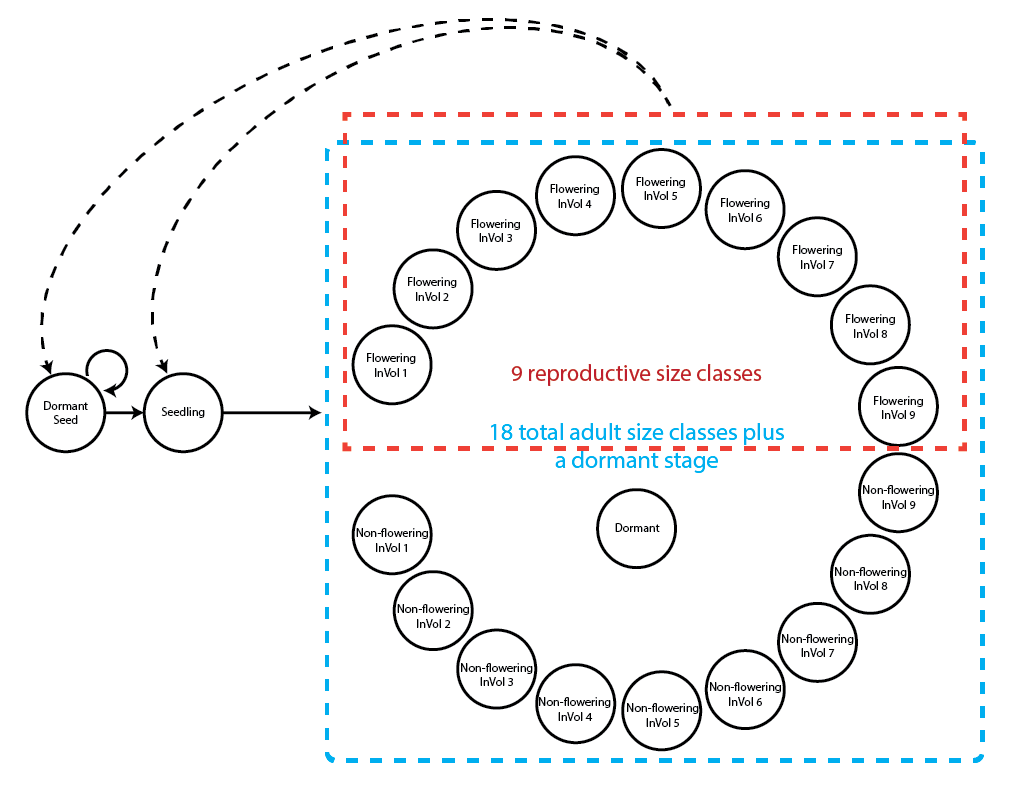Figure 8.1. Life history model of Lathyrus vernus using the log leaf volume as the size classification metric.

Our stageframe needs to include complete descriptions of all stages that occur in the dataset, with each stage defined uniquely, and also needs to describe for each stage portrayed in our life history model. Since this object can be used for automated classification of individuals, all sizes, reproductive states, and other characteristics defining each stage in the dataset need to be accounted for explicitly. This can be difficult if a few data points exist outside the range of sizes specified in the stageframe, because such situations generally lead to errors down the line. So, great care must be taken to include all size values and values of other descriptor variables occurring within the dataset. The final description of each stage occurring in the dataset must not completely overlap with any other stage also found in the dataset, although partial overlap is allowed and expected.

Before creating the stage frame, let’s explore the possible size variables. We will particularly look at summaries of the distribution of original and log sizes.

summary(c(lathyrus$Volume88, lathyrus$Volume89, lathyrus$Volume90, lathyrus$Volume91))
#>    Min. 1st Qu.  Median    Mean 3rd Qu.    Max.    NA's
#>     1.8    14.7   123.0   484.2   732.5  7032.0    1248
summary(c(lathyrus$lnVol88, lathyrus$lnVol89, lathyrus$lnVol90, lathyrus$lnVol91))
#>    Min. 1st Qu.  Median    Mean 3rd Qu.    Max.    NA's
#>   0.600   2.700   4.800   4.777   6.600   8.900    1248

The upper summary shows the original size, while the lower line shows the size given in logarithmic terms. We should note the size minima and maxima, because we have been using 0 as the size of vegetatively dormant individuals. The lowest uncorrected size is , with a maximum of . The minimum corrected size is , and the maximum corrected size is . Since the minimum corrected size is above 0 (i.e. all log sizes should be positive), and since the number of NAs has not increased (increased NAs would suggest that some unusable log sizes occur in the dataset), we are still able to use the log size value 0 as an indicator of vegetative dormancy. Note, however, that vegetative dormancy is also currently included in the many NAs that occur in size variables in this dataset.

It can also help to take a look at plots of these distributions. We will plot raw and log volume.

par(mfrow=c(1,2))
plot(density(c(lathyrus$Volume88, lathyrus$Volume89, lathyrus$Volume90, lathyrus$Volume91), na.rm = TRUE), main = "", xlab = "Volume", bty = "n")
plot(density(c(lathyrus$lnVol88, lathyrus$lnVol89, lathyrus$lnVol90, lathyrus$lnVol91), na.rm = TRUE), main = "", xlab = "Log volume", bty = "n")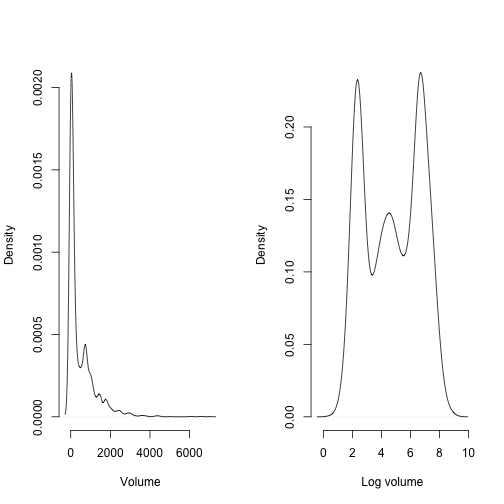Figure 8.2. Density plot of aboveground plant volume

Note how highly skewed the raw volume distribution is. This might cause some difficulty if we used raw size untransformed and with a Gaussian distribution. The log volume distribution looks ‘better’ than the raw volume distribution in the sense that it is closer to some semblance of a Gaussian distribution, mostly through an increased level of symmetry. This is helpful since our size metrics are decimals, and so cannot be treated as integers. If they could be treated as integers, then we could try modeling size as either Poisson- or negative binomial-distributed. We will work with log volume in this example, and treat it as Gaussian-distributed.

We will now develop a stageframe that incorporates the log volume of size. We will build this by creating vectors of the values describing each stage, always in the same order.

sizevector <- c(0, 4.6, 0, 1, 2, 3, 4, 5, 6, 7, 8, 9, 1, 2, 3, 4, 5, 6, 7, 8, 9)
stagevector <- c("Sd", "Sdl", "Dorm", "Sz1nr", "Sz2nr", "Sz3nr", "Sz4nr",
"Sz5nr","Sz6nr", "Sz7nr", "Sz8nr", "Sz9nr", "Sz1r", "Sz2r", "Sz3r", "Sz4r",
"Sz5r", "Sz6r", "Sz7r", "Sz8r", "Sz9r")
repvector <- c(0, 0, 0, 0, 0, 0, 0, 0, 0, 0, 0, 0, 1, 1, 1, 1, 1, 1, 1, 1, 1)
obsvector <- c(0, 1, 0, 1, 1, 1, 1, 1, 1, 1, 1, 1, 1, 1, 1, 1, 1, 1, 1, 1, 1)
matvector <- c(0, 0, 1, 1, 1, 1, 1, 1, 1, 1, 1, 1, 1, 1, 1, 1, 1, 1, 1, 1, 1)
immvector <- c(1, 1, 0, 0, 0, 0, 0, 0, 0, 0, 0, 0, 0, 0, 0, 0, 0, 0, 0, 0, 0)
propvector <- c(1, 0, 0, 0, 0, 0, 0, 0, 0, 0, 0, 0, 0, 0, 0, 0, 0, 0, 0, 0, 0)
indataset <- c(0, 1, 1, 1, 1, 1, 1, 1, 1, 1, 1, 1, 1, 1, 1, 1, 1, 1, 1, 1, 1)
binvec <- c(0, 4.6, 0.5, 0.5, 0.5, 0.5, 0.5, 0.5, 0.5, 0.5, 0.5, 0.5, 0.5, 0.5,
0.5, 0.5, 0.5, 0.5, 0.5, 0.5, 0.5)
minima <- c(0, 0, 1, 1, 1, 1, 1, 1, 1, 1, 1, 1, 1, 1, 1, 1, 1, 1, 1, 1, 1)
maxima <- c(NA, 1, NA, NA, NA, NA, NA, NA, NA, NA, NA, NA, NA, NA, NA, NA,
NA, NA, NA, NA, NA)
comments <- c("Dormant seed", "Seedling", "Dormant", "Size 1 Veg", "Size 2 Veg",
"Size 3 Veg", "Size 4 Veg", "Size 5 Veg", "Size 6 Veg", "Size 7 Veg",
"Size 8 Veg", "Size 9 Veg", "Size 1 Flo", "Size 2 Flo", "Size 3 Flo",
"Size 4 Flo", "Size 5 Flo", "Size 6 Flo", "Size 7 Flo", "Size 8 Flo",
"Size 9 Flo")
lathframeln <- sf_create(sizes = sizevector, stagenames = stagevector,
repstatus = repvector, obsstatus = obsvector, propstatus = propvector,
immstatus = immvector, matstatus = matvector, indataset = indataset,
binhalfwidth = binvec, minage = minima, maxage = maxima, comments = comments)
#lathframeln

#### Step 2a. Data organization

Once the stageframe is created, we can reorganize the dataset into historically-formatted vertical (hfv) format. Here, ‘vertical’ format is a way of organizing demographic data in which each row corresponds to the state of a single individual in two (if ahistorical) or three (if historical) consecutive monitoring occasions. To handle this, we will use the verticalize3() function, which creates historically-formatted vertical datasets, as below. We will also replace NAs in size and fecundity variables with 0s for modelsearch to work properly when we build models of vital rates, so we will now set NAas0 = TRUE. Some care needs to be taken with this last step, since some authors give missing values extra meaning not present in a value of 0. In our case, a missing value indicates that a plant was dead (both size and fecundity are missing), was alive but not sprouting (size was missing), or was alive but did not produce seed (fecundity was missing). In all cases, these NA values may be replaced by 0, because other variables indicate those conditions.

We also have two choices for use as our reproductive status and fecundity variables. The first choice, FCODE88 indicates whether a plant flowered. The second choice, Intactseed88, indicates the number of seed produced. The choice of which to use depends strongly on the aims of the study. In our case, we would like to treat all plants that flowered as reproductive, but treat fecundity in terms of real seed produced. The reason is that we believe that flowering plants have a different demography than non-flowering plants, either reflecting reproductive costs, or, conversely, because flowering plants might have more resources and hence higher survival than non-flowering plants, and so we wish to separate transitions among these two groups. So, let’s use FCODE88 to indicate reproductive status, and Intactseed88 to indicate fecundity. Once complete, we will look at a summary.

Finally, note that in the input to the following function, we utilize a strictly repeating pattern of variable names arranged in the same order for each monitoring occasion. This arrangement allows us to enter only the first variable in each set, as long as noyears and blocksize are set properly and no gaps or shuffles appear in the dataset. The data management functions that we have created for lefko3 do not require such repeating patterns, but they do make the required input in the function much shorter and more succinct.

lathvertln <- verticalize3(lathyrus, noyears = 4, firstyear = 1988,
patchidcol = "SUBPLOT", individcol = "GENET", blocksize = 9,
juvcol = "Seedling1988", sizeacol = "lnVol88", repstracol = "FCODE88",
nonobsacol = "Dormant1988", stageassign = lathframeln,
stagesize = "sizea", censorcol = "Missing1988", censorkeep = NA,
NAas0 = TRUE, censor = TRUE)
#summary(lathvertln)

Before we move on to the next key steps in analysis, let’s take a closer look at fecundity. In this dataset, fecundity is mostly a count of intact seeds, and only differs in six cases where the seed output was estimated based on other models. To see this, try the following code.

writeLines(paste0("Total length of variable corresponding to fecundity in year t+1: ", length(lathvertln$feca3))) #> Total length of variable corresponding to fecundity in year t+1: 2527 writeLines(paste0("Total non-integer entries in fecundity in year t+1: ", length(which(lathvertln$feca3 != round(lathvertln$feca3))))) #> Total non-integer entries in fecundity in year t+1: 0 writeLines(paste0("\nTotal length of variable corresponding to fecundity in year t: ", length(lathvertln$feca2)))
#>
#> Total length of variable corresponding to fecundity in year t: 2527
writeLines(paste0("Total non-integer entries in fecundity in year t: ", length(which(lathvertln$feca2 != round(lathvertln$feca2)))))
#> Total non-integer entries in fecundity in year t: 6
writeLines(paste0("\nTotal length of variable corresponding to fecundity in year t-1: ", length(lathvertln$feca1))) #> #> Total length of variable corresponding to fecundity in year t-1: 2527 writeLines(paste0("Total non-integer entries in fecundity in year t-1: ", length(which(lathvertln$feca1 != round(lathvertln$feca1))))) #> Total non-integer entries in fecundity in year t-1: 6 We see that we have quite a bit of fecundity data, and that it is overwhelmingly but not exclusively composed of integer values. The 6 non-integer values force us to make a decision - should we treat fecundity as a continuous variable, or round the values and treat it as a count variable? This is actually a vital decision that will strongly affect our vital rate model of fecundity, because treating it as continuous will also imply a Gaussian distribution. Let’s take a look at the distribution of values for this variable. plot(density(lathvertln$feca2), main = "", xlab = "Volume", bty = "n")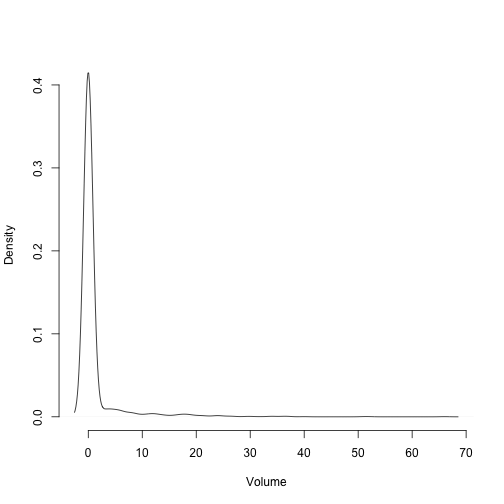Figure 8.3. Distribution of fecundity in time t in Lathyrus vernus

We see a distribution with a low mean value and a long right tail, suggesting strong skew and strong deviation from the Gaussian distribtuion. So, we will round fecundity so that we can treat fecundity as a count variable in the analysis.

lathvertln$feca3 <- round(lathvertln$feca3)
lathvertln$feca2 <- round(lathvertln$feca2)
lathvertln$feca1 <- round(lathvertln$feca1)

The fact that fecundity is now integer-based suggests that it can be treated as a count variable. This package currently allows 7 choices of count distributions: Gaussian, Poisson, negative binomial, zero-inflated Poisson, zero-inflated negative binomial, zero-truncated Poisson, and zero-truncated negative binomial. To assess which to use, we should first assess whether the mean and variance of the count are equal using a dispersion test. This test allows us to test whether the variance is greater than that expected under our mean value for fecundity using a chi-squared test. If it is not significantly different, then we may use some variant of the Poisson distribution. If the data are overdispersed, then we should use the negative binomial distribution. We should also test whether the number of zeroes is more than expected under these distributions, and make the distribution zero-inflated if so. Note that, because we have not excluded 0s from fecundity using reproductive status, we should not use a zero-truncated distribution.

Let’s start off by looking at a bar plot of the distribution of fecundity.

hist(subset(lathvertln, repstatus2 == 1)$feca2, main = "Fecundity", xlab = "Intact seeds produced in occasion t")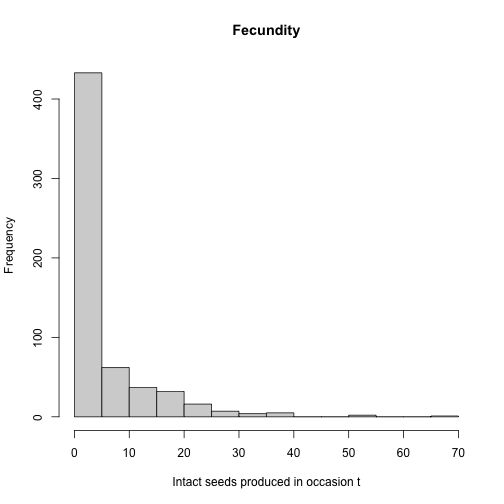Figure 8.4. Histogram of fecundity in occasion t We see that the distribution conforms to a classic count variable with a very low mean value. The first bar suggests that there may be too many zeroes, as well. Let’s now formally test our assumptions, that the mean equals the variance and that the number of zeroes meets expectation. Both tests use chi-squared distribution-based approaches, with the zero-inflation test in particular based on van der Broek (1995). sf_distrib(lathvertln, size2 = "sizea2", fec = "feca2", repst = "repstatus2") #> #> Mean fecundity is 4.791 #> The variance in fecundity is 70.14 #> The probability of this dispersion level by chance assuming the true mean fecundity = variance in fecundity, and an alternative hypothesis of overdispersion, is 0 #> #> Fecundity is significantly overdispersed. #> #> #> Mean lambda is 0.008302 #> The actual number of 0s in fecundity is 334 #> The expected number of 0s in fecundity under the null hypothesis is 4.973 #> The probability of this deviation in 0s is 0 #> #> Fecundity is significantly zero-inflated. #> NULL Such significant results for both tests show us that we really need to use a zero-inflated negative binomial distribution here. Now let’s move on to supplemental descriptive information. #### Step 2b. Provide supplemental information for matrix estimation Matrix estimation functions in package lefko3 are made to work with supplemental tables, which provide extra data for matrix estimation that is not included in the main demographic dataset. The supplemental() function provides a means of incorporating three additional kinds of data into MPM construction: 1. fixed transition values derived from other studies and added as constants to matrices, 2. proxy transition values when data for particular transitions do not exist and other, estimable transitions need to be used as proxies, and 3. reproductive multipliers indicating which stages lead to the production of which stages, and at what level relative to estimated fecundity. Here, we will create an ahistorical supplemental table organizing all of these sorts of data. Each row refers to a specific transition. The first 2 of these transitions are set to specific probabilities, which are the probabilities of germination and seed dormancy, estimated from a separate study. The final 2 terms are fecundity multipliers, which mark which transitions correspond to fecundity and provide information on what multiple of fecundity estimated via linear modeling applies to each case. Note that we can also include proxy transitions, in which we define a specific transition as being equal to another in the matrix. The latter approach is useful when some transitions cannot be estimated given a particular dataset, and so need to be set to other, proxy values that are estimable. lathsupp2 <- supplemental(stage3 = c("Sd", "Sdl", "Sd", "Sdl"), stage2 = c("Sd", "Sd", "rep", "rep"), givenrate = c(0.345, 0.054, NA, NA), multiplier = c(NA, NA, 0.345, 0.054), type = c(1, 1, 3, 3), stageframe = lathframeln, historical = FALSE) #lathsupp2 #### Step 3. Tests of history, and vital rate modeling Next we will run the modelsearch function with the new vertical dataset. This function will develop our best-fit vital rate models for us. This function looks simple, but it automates several crucial and complex tasks in MPM analysis. Specifically, it automates 1) the building of global models for each vital rate requested, 2) the exhaustive construction of all reduced models, and 3) the selection of the best-fit models. Let’s look at some of the options that we will utilize for this purpose (please note that this list includes only some of the options actually offered by the function - further options are shown in the documentation for modelsearch(), with further theoretical details shown in the Basic theory and concepts vignette and example uses in the other vignettes). historical: Setting this to TRUE or FALSE indicates to include state in occasion t-1 in the global models. approach: Designates whether to use generalized linear models (glm), in which all factors are treated as fixed, or mixed effects models (mixed), in which factors are treated as either fixed or random (most notably, time, patch, and individual identity can be treated as random). suite: Designates which factors to include in the global model. Possible values include size, which includes size only; rep, which includes reproductive status only; main, which includes both size and reproductive status as main effects only; full, which includes both size and reproductive status and all two-way interactions; and const, which includes only an intercept. These factors are in addition to individual identity, patch, and time. vitalrates: Designates which vital rates to model. The default is to model survival, size, and fecundity, but users can also model observation status and reproduction status or drop some terms. juvestimate: Designates the name of the juvenile stage transitioning to maturity, in cases where the dataset includes data on juveniles. juvsize: Denotes whether size should be used as an independent term in models involving transition from the juvenile stage. bestfit: Denotes whether the best-fit model should be chosen as the model with the lowest AICc (AICc) or as the most parsimonious model (AICc&k), where the latter is the model that has the fewest estimated parameters and is within 2 AICc units of the model with the lowest AICc. sizedist: Designates the distribution used for size. The options include gaussian, poisson, and negbin, the last of which refers to the negative binomial distribution (quadratic parameterization). fecdist: Designates the distribution used for fecundity. The options include gaussian, poisson, and negbin, the last of which refers to the negative binomial distribution (quadratic parameterization). fectime: Designates whether fecundity is estimated within occasion t (the default) or occasion t+1. Plant ecologists are likely to choose the former, since fecundity is typically estimated via a proxy such as flowers, fruit, or seed produced. Wildlife ecologists might choose the latter, since fecundity may be best estimated as a count of actual juveniles within nests, burrows, or other family structures. size.zero: Designates whether the size distribution should be zero-inflated. Only applies to the Poisson and negative binomial distributions. size.trunc: Designates whether to use a zero-truncated distribution for size. Only applies to the Poisson and negative binomial distributions, and cannot be applied if size.zero = TRUE. fec.zero: Designates whether the fecundity distribution should be zero-inflated. Only applies to the Poisson and negative binomial distributions. fec.trunc: Designates whether to use a zero-truncated distribution for fecundity. Only applies to the Poisson and negative binomial distributions, and cannot be applied if fec.zero = TRUE. jsize.zero: Designates whether the juvenile size distribution should be zero-inflated. Only applies to the Poisson and negative binomial distributions. jsize.trunc: Designates whether to use a zero-truncated distribution for juvenile size. Only applies to the Poisson and negative binomial distributions, and cannot be applied if jsize.zero = TRUE. indiv: Designates the variable corresponding to individual identity in the vertical dataset. patch: Designates the variable corresponding to patch identity in the vertical dataset. year: Designates the variable corresponding to occasion t in the vertical dataset. age: Designates the name of the variable corresponding to age in occasion t. Should be set only if an age-by-stage model is desired. Do not use this option to produce a model that does not incorporate age. year.as.random: Designates whether to treat year as a random factor. Only used when a mixed effects modeling approach is chosen (approach = "mixed"). patch.as.random: Designates whether to treat patch identity as a random factor. Only used when a mixed effects modeling approach is chosen (approach = "mixed"). show.model.tables: Designates whether to include the full dredge model tables showing all models developed and their associated AICc values. quiet: Denotes whether to silence most guidepost statements and warning messages during vital rate modeling. If TRUE, then only warnings incurred in model testing will be visible, including warnings from the testing of global models and those from the testing of reduced models produced during model dredging. In addition, there are other options that provide flexibility in handling datasets with different designations for key variables, and allowing manual designation of stages. Here, we will create two ahistorical model sets. The first will be a model set for the entire population, without separating patches. The second will include patch as a random factor, and will thus allow us to explore patch dynamics as well as population dynamics. We will not create a historical set this time because we are producing an age x stage MPM only - lefko3 does not currently estimate or support historical age x stage MPMs. lathmodelsln2 <- modelsearch(lathvertln, historical = FALSE, approach = "mixed", suite = "main", vitalrates = c("surv", "obs", "size", "repst", "fec"), juvestimate = "Sdl", bestfit = "AICc&k", sizedist = "gaussian", fecdist = "negbin", indiv = "individ", year = "year2", age = "obsage", year.as.random = TRUE, patch.as.random = TRUE, show.model.tables = TRUE, fec.zero = TRUE, quiet = TRUE) #> boundary (singular) fit: see ?isSingular #> boundary (singular) fit: see ?isSingular #> boundary (singular) fit: see ?isSingular lathmodelsln2p <- modelsearch(lathvertln, historical = FALSE, approach = "mixed", suite = "main", vitalrates = c("surv", "obs", "size", "repst", "fec"), juvestimate = "Sdl", bestfit = "AICc&k", sizedist = "gaussian", fecdist = "negbin", indiv = "individ", patch = "patchid", year = "year2", age = "obsage", year.as.random = TRUE, patch.as.random = TRUE, show.model.tables = TRUE, fec.zero = TRUE, quiet = TRUE) #> boundary (singular) fit: see ?isSingular #> boundary (singular) fit: see ?isSingular #> boundary (singular) fit: see ?isSingular #> boundary (singular) fit: see ?isSingular #> boundary (singular) fit: see ?isSingular #summary(lathmodelsln2) #summary(lathmodelsln2p) The output can be rather verbose, and so we have limited it with the quiet = TRUE option. The function was developed to provide text marker posts of what the function is doing and what it has accomplished, as well as to show all warnings from all workhorse functions used. Because we used the mixed approach here, this includes warnings originating from estimating mixed linear models with package lme4 (Bates et al. 2015) and, in the case of fecundity, the package glmmTMB (Brooks et al. 2017). It also shows warnings originating from the dredge() function of package MuMIn (Bartoń 2014), which is the core function used in model building and AICc estimation. We encourage users to get familiar with interpreting these warnings and assessing the degree to which they impact their own analyses. In developing these linear models, the modelsearch() function created a series of nested datasets to estimate vital rates as conditional rates and probabilities. The exact subsets created depend on which parameters were called for. It is important to consider which are required, and particular attention needs to be paid if there are size classes with sizes of 0. In these situations, it is best to consider a size of 0 as unobservable, and so to introduce observation status as a vital rate to absorb these sizes. This sets up datasets for size estimation that do not include 0 in the response term, because all 0 responses are already absorbed by observation status. A look at the summaries shows that the best-fit models vary in complexity. Age is important in four of the adult vital rates, in both the population-only model set as well as the patch model set. For example, survival is influenced by reproductive status and age in the current year, as well as by patch, individual identity, and year, while observation status is not influenced by age. We can see these models explicitly, as well as the model tables developed, by calling them directly from the lefkoMod object. #### Step 4. MPM estimation Next, we will estimate the ahistorical sets of matrices. We will match the ahistorical age-by-stage matrix estimation function, aflefko2(), with the appropriate ahistorical input, including the ahistorical lefkoMod objects lathmodelsln2 and lathmodelsln2p. Model sets that include historical terms should not be used to create ahistorical matrices, since the coefficients in the best-fit models are estimated assuming a specific model structure that either relies on historical terms or does not. Historical vital rate models may yield biased results if used to construct ahistorical matrices. Also note that lefko3 does not currently allow the construction of historical age-by-stage MPMs. lathmat2age <- aflefko2(year = "all", stageframe = lathframeln, modelsuite = lathmodelsln2, data = lathvertln, supplement = lathsupp2, patchcol = "patchid", yearcol = "year2", year.as.random = FALSE, patch.as.random = FALSE, final_age = 2, continue = TRUE, reduce = FALSE) lathmat2agep <- aflefko2(year = "all", patch = "all", stageframe = lathframeln, modelsuite = lathmodelsln2p, data = lathvertln, supplement = lathsupp2, patchcol = "patchid", yearcol = "year2", year.as.random = FALSE, patch.as.random = FALSE, final_age = 2, continue = TRUE, reduce = FALSE) summary(lathmat2age) #> #> This ahistorical lefkoMat object contains 3 matrices. #> #> Each matrix is a square matrix with 63 rows and columns, and a total of 3969 elements. #> A total of 2178 survival transitions were estimated, with 726 per matrix. #> A total of 108 fecundity transitions were estimated, with 36 per matrix. #> #> Vital rate modeling quality control: #> #> Survival estimated with 257 individuals and 2246 individual transitions. #> Observation estimated with 254 individuals and 2121 individual transitions. #> Size estimated with 254 individuals and 1916 individual transitions. #> Reproductive status estimated with 254 individuals and 1916 individual transitions. #> Fecundity estimated with 128 individuals and 599 individual transitions. #> Juvenile survival estimated with 187 individuals and 281 individual transitions. #> Juvenile observation estimated with 154 individuals and 210 individual transitions. #> Juvenile size estimated with 144 individuals and 193 individual transitions. #> Juvenile reproduction probability not estimated. #> #> Survival probability sum check (each matrix represented by column in order): #> [,1] [,2] [,3] #> Min. 0.000 0.000 0.000 #> 1st Qu. 0.000 0.000 0.000 #> Median 0.960 0.949 0.909 #> Mean 0.604 0.603 0.588 #> 3rd Qu. 0.985 0.982 0.967 #> Max. 0.997 0.995 0.990 #> NULL summary(lathmat2agep) #> #> This ahistorical lefkoMat object contains 18 matrices. #> #> Each matrix is a square matrix with 63 rows and columns, and a total of 3969 elements. #> A total of 13068 survival transitions were estimated, with 726 per matrix. #> A total of 648 fecundity transitions were estimated, with 36 per matrix. #> #> Vital rate modeling quality control: #> #> Survival estimated with 257 individuals and 2246 individual transitions. #> Observation estimated with 254 individuals and 2121 individual transitions. #> Size estimated with 254 individuals and 1916 individual transitions. #> Reproductive status estimated with 254 individuals and 1916 individual transitions. #> Fecundity estimated with 128 individuals and 599 individual transitions. #> Juvenile survival estimated with 187 individuals and 281 individual transitions. #> Juvenile observation estimated with 154 individuals and 210 individual transitions. #> Juvenile size estimated with 144 individuals and 193 individual transitions. #> Juvenile reproduction probability not estimated. #> #> Survival probability sum check (each matrix represented by column in order): #> [,1] [,2] [,3] [,4] [,5] [,6] [,7] [,8] [,9] [,10] [,11] [,12] [,13] [,14] [,15] [,16] #> Min. 0.000 0.000 0.000 0.000 0.000 0.000 0.000 0.000 0.000 0.000 0.000 0.000 0.000 0.000 0.000 0.000 #> 1st Qu. 0.000 0.000 0.000 0.000 0.000 0.000 0.000 0.000 0.000 0.000 0.000 0.000 0.000 0.000 0.000 0.000 #> Median 0.966 0.957 0.924 0.959 0.948 0.906 0.960 0.956 0.918 0.958 0.935 0.887 0.961 0.939 0.894 0.963 #> Mean 0.607 0.606 0.594 0.603 0.602 0.586 0.604 0.604 0.591 0.605 0.599 0.581 0.606 0.600 0.583 0.605 #> 3rd Qu. 0.987 0.985 0.973 0.984 0.982 0.966 0.986 0.984 0.970 0.983 0.980 0.964 0.984 0.981 0.966 0.985 #> Max. 0.997 0.996 0.992 0.997 0.994 0.990 0.997 0.995 0.991 0.996 0.994 0.988 0.996 0.994 0.989 0.997 #> [,17] [,18] #> Min. 0.000 0.000 #> 1st Qu. 0.000 0.000 #> Median 0.950 0.910 #> Mean 0.603 0.588 #> 3rd Qu. 0.983 0.968 #> Max. 0.995 0.990 #> NULL The first model set led to the development of 3 matrices, reflecting the 4 years of data. The second model set led to the development of 18 matrices, reflecting 4 years and 6 patches. The quality control section gives us a sense of the amount of data used to model each vital rate, and also shows us that the survival-transition (U) matrices are composed entirely of proper probabilities yielding stage survival probability falling between 0.0 and 1.0. Matrix estimation can sometimes create spurious values, such as stage survival greater than 1.0. Such values can occur for a variety of reasons, but the most common is the inclusion through a supplemental table or overwrite table of externally-determined survival probabilities that are too high. Make sure to check your matrix column sums each time you estimate MPMs to prevent this problem. Survival greater than 1.0 can lead to strange effects on metrics of population dynamics. We can get a sense of what these matrices look like by visualizing them. Let’s use the image3() function to look at just one. image3(lathmat2age, used = 1)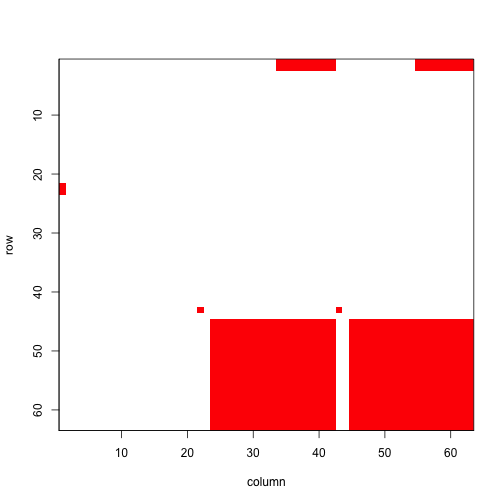Figure 8.5. Visualization of 1st A Matrix #> NULL The clear squares refer to zero elements, and the red elements refer to non-zero values corresponding to survival transitions and fecundity. The vast number of 0s may be surprising, but this matrix is a supermatrix organized by age first, with stage organizing within-age blocks. The first age is age 0, which cannot be adult, and age 1 corresponds to seedlings, leading to most non-zero elements in the adult portion. The adult block occurs from age 2, and this block can perpetuate indefinitely. The number of elements estimated is greater now than in the typical ahistorical MPM, because now we have added age as a major factor for analysis. This matrix is overwhelmingly composed of elements that must be 0, and so it is a rather sparse matrix ((726 + 36) / 3969 = 19.2% of elements). We can see the order of ages and stages using the agestages element of the lefkoMat object we produced, as below. Note that our matrix is 63 rows by 63 columns, and this object gives us the exact order used. #lathmat2age$agestages

Now let’s estimate the element-wise arithmetic mean matrices. The first lefkoMat object created will include a single mean matrix, while the second will include 6 mean matrices for the patches, followed by a grand mean matrix, yielding a total of 7 matrices.

lathmat2mean <- lmean(lathmat2age)
lathmat2pmean <- lmean(lathmat2agep)

summary(lathmat2mean)
#>
#> This ahistorical lefkoMat object contains 1 matrix.
#>
#> Each matrix is a square matrix with 63 rows and columns, and a total of 3969 elements.
#> A total of 726 survival transitions were estimated, with 726 per matrix.
#> A total of 36 fecundity transitions were estimated, with 36 per matrix.
#>
#> Survival probability sum check (each matrix represented by column in order):
#>          [,1]
#> Min.    0.000
#> 1st Qu. 0.000
#> Median  0.941
#> Mean    0.598
#> 3rd Qu. 0.973
#> Max.    0.993
#> NULL
summary(lathmat2pmean)
#>
#> This ahistorical lefkoMat object contains 7 matrices.
#>
#> Each matrix is a square matrix with 63 rows and columns, and a total of 3969 elements.
#> A total of 5082 survival transitions were estimated, with 726 per matrix.
#> A total of 252 fecundity transitions were estimated, with 36 per matrix.
#>
#> Survival probability sum check (each matrix represented by column in order):
#>          [,1]  [,2]  [,3]  [,4]  [,5]  [,6]  [,7]
#> Min.    0.000 0.000 0.000 0.000 0.000 0.000 0.000
#> 1st Qu. 0.000 0.000 0.000 0.000 0.000 0.000 0.000
#> Median  0.952 0.938 0.947 0.932 0.936 0.942 0.940
#> Mean    0.602 0.597 0.599 0.595 0.597 0.599 0.598
#> 3rd Qu. 0.978 0.971 0.975 0.973 0.974 0.974 0.973
#> Max.    0.994 0.993 0.994 0.992 0.992 0.993 0.993
#> NULL

#### Step 5. MPM analysis

Now let’s estimate the deterministic population growth rate $$\lambda$$, and the stochastic population growth rate, $$a = \text{log} \lambda _{S}$$, in our age x stage MPM. Let’s plot these for comparison, making sure to take the natural exponent of the stochastic growth rate to make it comparable to $$\lambda$$. We will show the patch means and the grand population mean.

lambda2 <- lambda3(lathmat2age)
lambda2m <- lambda3(lathmat2mean)
lambda2mp <- lambda3(lathmat2pmean)
set.seed(42)
sl2 <- slambda3(lathmat2age) #Stochastic growth rate
sl2$expa <- exp(sl2$a)

par(mfrow = c(1,2))
plot(lambda ~ year2, data = lambda2, ylim = c(0.92, 0.98),xlab = "Year",
ylab = expression(lambda), type = "l", lty= 1, lwd = 2, bty = "n")
abline(a = lambda2m$lambda, b = 0, lty = 2, lwd= 2, col = "orangered") abline(a = sl2$expa, b = 0, lty = 2, lwd= 3, col = "darkred")
legend("bottomright", c("det annual", "det mean", "stochastic"), lty = c(1, 2, 2),
col = c("black", "orangered", "darkred"), lwd = c(2, 2, 3), bty = "n")

plot(lambda ~ patch, data = lambda2mp[1:6,], ylim = c(0.92, 0.98), xlab = "Patch",
ylab = expression(lambda), type = "l", lty= 1, lwd = 2, bty = "n")
abline(a = lambda2m$lambda, b = 0, lty = 2, lwd= 2, col = "orangered") abline(a = sl2$expa, b = 0, lty = 2, lwd= 2, col = "darkred")
legend("bottomleft", c("patch det mean", "pop det mean", "pop sto"), lty = c(1, 2, 2),
col = c("black", "orangered", "darkred"), lwd = 2, bty = "n")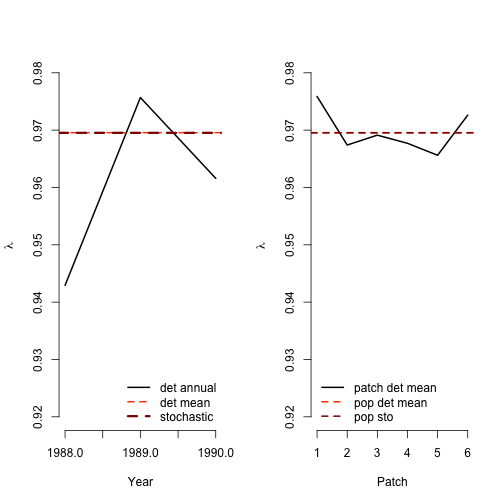Figure 8.6. Deterministic vs. stochastic lambda

Deterministic and stochastic analyses suggest that the population and all patches are declining, with all years and patches associated with $$\lambda < 1$$ and $$a = \text{log} \lambda _{S} < 0$$. Qualitatively, they agree with the $$\lambda$$ and $$a = \text{log} \lambda _{S}$$ estimates from our other Lathyrus vignettes.

Now let’s look at the stable stage distribution, using the population matrices rather than the patch matrices. The output for the ahistorical MPM is a dataframe with matrix of origin, stage name, and stable stage proportion in each row. Note that the ss_prop column, which gives us the stable stage proportion of each stage, sums to 1.0 within each matrix.

ehrlen2ss <- stablestage3(lathmat2mean)
ehrlen2ss_s <- stablestage3(lathmat2age, stochastic = TRUE, seed = 42)

ss_props <- cbind.data.frame(ehrlen2ss$ss_prop, ehrlen2ss_s$ss_prop)
names(ss_props) <- c("det", "sto")
rownames(ss_props) <- paste(ehrlen2ss$age, ehrlen2ss$stage)

barplot(t(ss_props), beside = T, ylab = "Proportion", xlab = "Age-Stage",
ylim = c(0, 0.25), xaxt = "n", col = c("black", "red"), bty = "n")
text(cex=0.5, y = -0.015, x = seq(from = 0, to = 2.98*length(rownames(ss_props)),
by = 3), rownames(ss_props), xpd=TRUE, srt=45)
legend("topright", c("deterministic", "stochastic"), col = c("black", "red"),
pch = 15, bty = "n")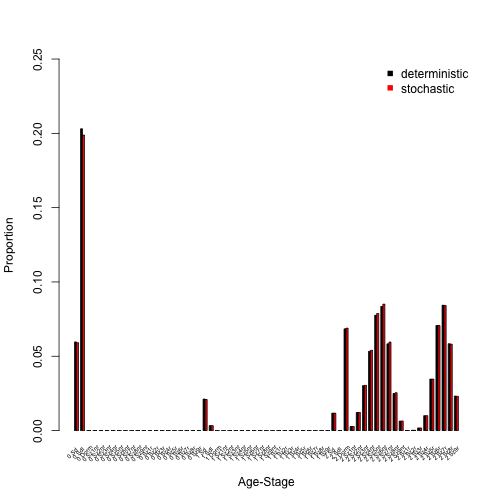Figure 8.7. Stable and long-run age-stage distribution

The distribution is actually a stable age-stage distribution, and the deterministic and stochastic approaches generally agree. The population is dominated by seedlings, dormant seeds, and mid-sized adults (both flowering and non-flowering). To explore further, it may be useful to collapse age and only look at stage, as below.

det_dist <- apply(as.matrix(c(1:21)), 1, function(X) {
ss_sum <- ss_props$det[X] + ss_props$det[21 + X] + ss_props$det[42 + X] return(ss_sum) }) sto_dist <- apply(as.matrix(c(1:21)), 1, function(X) { ss_sum <- ss_props$sto[X] + ss_props$sto[21 + X] + ss_props$sto[42 + X]
return(ss_sum)
})

barplot(t(cbind.data.frame(det_dist, sto_dist)), beside = T,  ylab = "Proportion",
xaxt = "n", ylim = c(0, 0.25), col = c("black", "red"), bty = "n")
text(cex=1, x=seq(from = 0.5, to = 3 * length(lathframeln$stage), by = 3), y=-0.025, lathframeln$stage, xpd=TRUE, srt=45)
legend("topright", c("deterministic", "stochastic"), col = c("black", "red"),
pch = 15, bty = "n")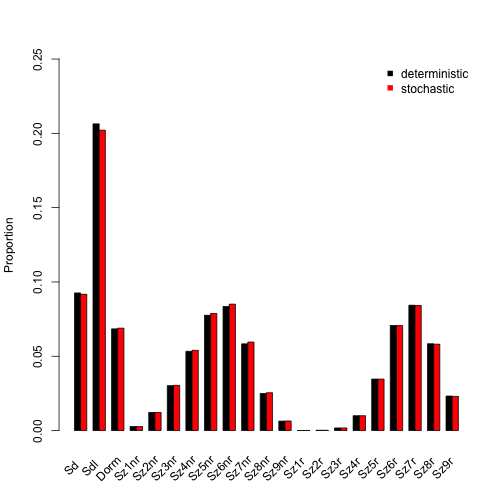Figure 8.8. Stable and long-run stage distribution

The stable stage distribution shows very high levels of seedlings and dormant seeds, and also of non-flowering and flowering mid-size adults. So, we see the same patterns as before, but without age to make us squint at the x-axis labels.

Now let’s take look at the reproductive values. We’ll go straight to the plots, as with the stable stage distribution.

ehrlen2rv <- repvalue3(lathmat2mean)
ehrlen2rv_s <- repvalue3(lathmat2age, stochastic = TRUE, seed = 42)

barplot(t(cbind.data.frame(ehrlen2rv$rep_value, ehrlen2rv_s$rep_value)),
beside = T, ylab = "Relative rep value", xlab = "Age-Stage", ylim = c(0, 1.3),
col = c("black", "red"), bty = "n")
text(cex=0.5, y = -0.06, x = seq(from = 0, to = 2.98*length(rownames(ss_props)),
by = 3), rownames(ss_props), xpd=TRUE, srt=45)
legend("topleft", c("deterministic", "stochastic"), col = c("black", "red"),
pch = 15, bty = "n")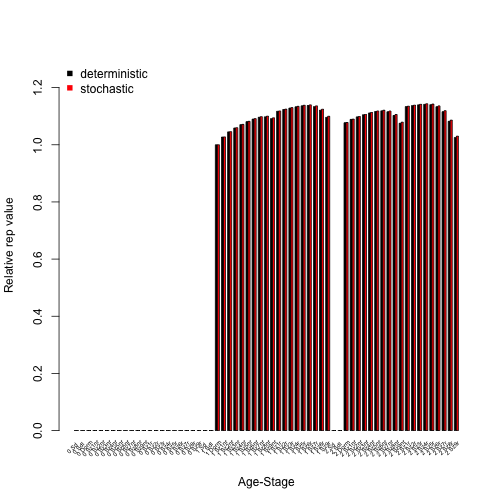Figure 8.9. Age-stage reproductive values

We see reproductive values above 0 starting with non-reproductive adults in age 1, and the highest reproductive values associated with flowering, medium to large adults in ages 1 and 2. These patterns hold in both deterministic and stochastic analyses.

We will next assess to which matrix elements $$\lambda$$ is most sensitive and elastic to changes in. As before, we will look at both deterministic and stochastic sensitivities.

lathmat2msens <- sensitivity3(lathmat2mean)
set.seed(42)
lathmat2msens_s <- sensitivity3(lathmat2age, stochastic = TRUE)

writeLines("\nThe highest deterministic sensitivity value: ")
#>
#> The highest deterministic sensitivity value:
max(lathmat2msens$ah_sensmats[][which(lathmat2mean$A[] > 0)])
#>  0.1239382
writeLines("\nThis value is associated with element: ")
#>
#> This value is associated with element:
which(lathmat2msens$ah_sensmats[] == max(lathmat2msens$ah_sensmats[][which(lathmat2mean$A[] > 0)])) #>  3838 writeLines("\nThe highest deterministic sensitivity value: ") #> #> The highest deterministic sensitivity value: max(lathmat2msens_s$ah_sensmats[][which(lathmat2mean$A[] > 0)]) #>  0.1288112 writeLines("\nThis value is associated with element: ") #> #> This value is associated with element: which(lathmat2msens_s$ah_sensmats[] == max(lathmat2msens_s$ah_sensmats[][which(lathmat2mean$A[] > 0)]))
#>  3838

The highest sensitivity value in both analyses appears to be associated with the transition from flowering adult age 2 and up in size class 7, to flowering adult age 2 and up in size class 4 (element 3838, in column 61, row 58). Inspecting the sensitivity matrix (type lathmat2msens$ah_sensmats[] to view the full deterministic sensitivity matrix, or lathmat2msens_s$ah_sensmats[] to view the full stochastic sensitivity matrix) also shows that transitions near that element in the matrix are associated with rather high sensitivities.

Let’s now assess the elasticity of $$\lambda$$ or $$\text{log} \lambda$$ to matrix elements.

lathmat2melas <- elasticity3(lathmat2mean)
set.seed(42)
lathmat2melas_s <- elasticity3(lathmat2age, stochastic = TRUE)

writeLines("\nThe highest deterministic elasticity value: ")
#>
#> The highest deterministic elasticity value:
max(lathmat2melas$ah_elasmats[][which(lathmat2mean$A[] > 0)])
#>  0.02815572
writeLines("\nThe largest determnistic elasticity is associated with element: ")
#>
#> The largest determnistic elasticity is associated with element:
which(lathmat2melas$ah_elasmats[] == max(lathmat2melas$ah_elasmats[]))
#>  3841
writeLines("\nThe highest stochastic elasticity value: ")
#>
#> The highest stochastic elasticity value:
max(lathmat2melas_s$ah_elasmats[][which(lathmat2mean$A[] > 0)])
#>  0.02841286
writeLines("\nThe largest stochastic elasticity is associated with element: ")
#>
#> The largest stochastic elasticity is associated with element:
which(lathmat2melas_s$ah_elasmats[] == max(lathmat2melas_s$ah_elasmats[]))
#>  3841

Both deterministic and stochastic analyses show that population growth rate is most elastic to element 3841, which is in column 61 and row 61. Checking our stageframe shows that this element is associated with stasis in flowering adult age 2 and up in size class 7.

Elasticity values are additive and sum to 1.0 within single matrices. This allows us to make interesting comparisons, such as to compare the elasticity of population growth rate to changes in transitions associated with specific stages. Let’s conduct such an analysis, and plot the results.

elas_put_together <- cbind.data.frame(colSums(lathmat2melas$ah_elasmats[]), colSums(lathmat2melas_s$ah_elasmats[]))
names(elas_put_together) <- c("det", "sto")
rownames(elas_put_together) <- apply(as.matrix(c(1:dim(lathmat2melas$agestages))), 1, function(X) { paste(lathmat2melas$agestages$stage[X], lathmat2melas$agestages$age[X]) }) barplot(t(elas_put_together), beside=T, names.arg = rep(NA, length(rownames(elas_put_together))), ylab = "Elasticity", xlab = "Stage", col = c("black", "orangered"), bty = "n") text(cex=0.5, y = -0.007, x = seq(from = 0, to = 2.98*length(rownames(elas_put_together)), by = 3), rownames(elas_put_together), xpd=TRUE, srt=45) legend("topleft", c("deterministic", "stochastic"), col = c("black", "orangered"), pch = 15, bty = "n")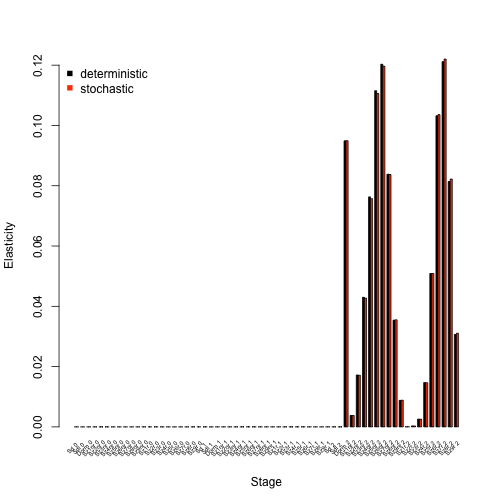Figure 8.10. Elasticities by age-stage We see in the analysis above that in both the deterministic and stochastic cases, the population growth rate is most elastic to changes associated with flowering and non-flowering mid-sized adults, and with vegetatively dormant adults. Juvenile stages have little influence. Our estimated elasticities can also be summarized by transition type. Let’s take a look at a plot, using the summary.lefkoElas() function to help us assess these transition types. lathmat2m_sums <- summary(lathmat2melas) lathmat2m_s_sums <- summary(lathmat2melas_s) elas_sums_together <- cbind.data.frame(lathmat2m_sums$ahist[,2],
lathmat2m_s_sums$ahist[,2]) names(elas_sums_together) <- c("det", "sto") rownames(elas_sums_together) <- lathmat2m_sums$ahist$category barplot(t(elas_sums_together), beside=T, ylab = "Elasticity", xlab = "Transition", col = c("black", "orangered"), bty = "n") legend("topright", c("deterministic", "stochastic"), col = c("black", "orangered"), pch = 15, bty = "n")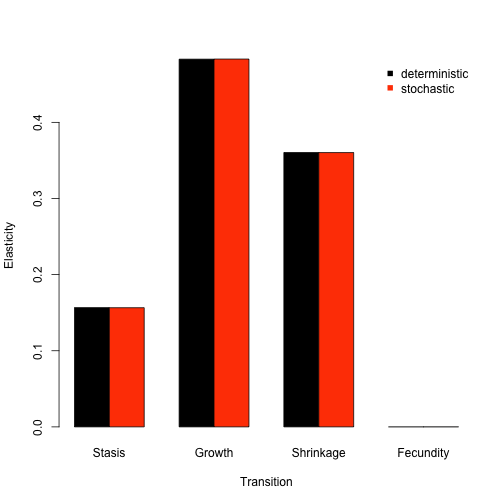Figure 8.11. Elasticities by transition type We see in the plot above that deterministic and stochastic analyses agree strongly about the importance and influence of transition types to population growth rate. Specifically, the population growth rate is most strongly elastic in response to changes in growth transitions, and completely inelastic to changes in fecundity. Shrinkage is also fairly important and influential. In addition to sensitivity and elasticity analyses, we can use package lefko3 to assess the actual impacts of demographic shifts or differences on the population growth rate. Two tools for this purpose are the life table response experiment (LTRE), and its stochastic version the stochastic life table response experiment (sLTRE). Both are available via the ltre3() function. Below, we perform a standard deterministic LTRE to assess the impact of space on the population growth rate. This is done via a comparison of patch-level demography to the grand mean matrix, which is the default comparison if no reference matrices are provided. Remove the hashtag from the second line to see the full structure of the resulting object. Particularly, you will notice that the first element, ltre_det, is a list of 6 matrices. These 6 matrices show the actual impact of the difference in elements between the respective patch-level matrix and the reference matrix (here, the grand population mean matrix) on the population growth rate $$\lambda$$. After this, we see similar structure to the input lefkoMat object, including the stageframe and order of matrices. lathmat2m_ltre <- ltre3(lathmat2pmean) #> Warning: Matrices input as mats will also be used as reference matrices. #> Using all refmats matrices as reference matrices. #lathmat2m_ltre The sLTRE produces output that is a bit different. Run the code below, removing the hashtag to see the output. Here, we see two lists of matrices prior to the MPM metadata. The first, ltre_mean, is a list of matrices showing the impact of differences in mean elements between the patch-level temporal mean matrices and the reference temporal mean matrix. The second, ltre_sd, is a list of matrices showing the impact of differences in the temporal standard deviation of each element between the patch-level and reference matrix sets. In other words, while a standard LTRE shows the impact of changes in matrix elements on $$\lambda$$, the sLTRE shows the impacts of changes in the mean and the variability of matrix elements on $$\text{log} \lambda$$. lathmat2m_sltre <- ltre3(lathmat2agep, stochastic = TRUE, rseed = 42) #> Warning: Matrices input as mats will also be used as reference matrices. #> Using all refmats matrices as reference matrices. #lathmat2m_sltre The output objects are large and can take a great deal of effort to look over and understand. Therefore, we will show three approaches to assessing these objects, using an approach similar to that used to assess elasticities. These methods can be used to assess patterns in all 6 patches, but for brevity we will focus only on the first matrix here. First, we will identify the elements most strongly impacting the population growth rate in each case. writeLines("The greatest (i.e most positive) deterministic LTRE contribution: ") #> The greatest (i.e most positive) deterministic LTRE contribution: max(lathmat2m_ltre$ltre_det[])
#>  0.001885109
writeLines("The greatest deterministic LTRE contribution is associated with element: ")
#> The greatest deterministic LTRE contribution is associated with element:
which(lathmat2m_ltre$ltre_det[] == max(lathmat2m_ltre$ltre_det[]))
#>  3841
writeLines("The lowest (i.e. most negative) deterministic LTRE contribution: ")
#> The lowest (i.e. most negative) deterministic LTRE contribution:
min(lathmat2m_ltre$ltre_det[]) #>  -0.003198877 writeLines("The lowest deterministic LTRE contribution is associated with element: ") #> The lowest deterministic LTRE contribution is associated with element: which(lathmat2m_ltre$ltre_det[] == min(lathmat2m_ltre$ltre_det[])) #>  3825 writeLines("\nThe greatest (i.e most positive) stochastic mean LTRE contribution: ") #> #> The greatest (i.e most positive) stochastic mean LTRE contribution: max(lathmat2m_sltre$ltre_mean[])
#>  0.001732296
writeLines("The greatest stochastic mean LTRE contribution is associated with element: ")
#> The greatest stochastic mean LTRE contribution is associated with element:
which(lathmat2m_sltre$ltre_mean[] == max(lathmat2m_sltre$ltre_mean[]))
#>  3841
writeLines("The lowest (i.e. most negative) stochastic mean LTRE contribution: ")
#> The lowest (i.e. most negative) stochastic mean LTRE contribution:
min(lathmat2m_sltre$ltre_mean[]) #>  -0.003708382 writeLines("The lowest stochastic mean LTRE contribution is associated with element: ") #> The lowest stochastic mean LTRE contribution is associated with element: which(lathmat2m_sltre$ltre_mean[] == min(lathmat2m_sltre$ltre_mean[])) #>  3195 writeLines("\nThe greatest (i.e most positive) stochastic SD LTRE contribution: ") #> #> The greatest (i.e most positive) stochastic SD LTRE contribution: max(lathmat2m_sltre$ltre_sd[])
#>  3.938314e-05
writeLines("The greatest stochastic SD LTRE contribution is associated with element: ")
#> The greatest stochastic SD LTRE contribution is associated with element:
which(lathmat2m_sltre$ltre_sd[] == max(lathmat2m_sltre$ltre_sd[]))
#>  3138
writeLines("The lowest (i.e. most negative) stochastic SD LTRE contribution: ")
#> The lowest (i.e. most negative) stochastic SD LTRE contribution:
min(lathmat2m_sltre$ltre_sd[]) #>  -3.324041e-05 writeLines("The lowest stochastic SD LTRE contribution is associated with element: ") #> The lowest stochastic SD LTRE contribution is associated with element: which(lathmat2m_sltre$ltre_sd[] == min(lathmat2m_sltre$ltre_sd[])) #>  3843 writeLines("\nTotal positive contribution of shifts in deterministic LTRE contributions: ") #> #> Total positive contribution of shifts in deterministic LTRE contributions: sum(lathmat2m_ltre$ltre_det[][which(lathmat2m_ltre$ltre_det[] > 0)]) #>  0.03701323 writeLines("Total negative contribution of shifts in deterministic LTRE contributions: ") #> Total negative contribution of shifts in deterministic LTRE contributions: sum(lathmat2m_ltre$ltre_det[][which(lathmat2m_ltre$ltre_det[] < 0)]) #>  -0.03156971 writeLines("\nTotal positive contribution of shifts in stochastic mean LTRE contributions: ") #> #> Total positive contribution of shifts in stochastic mean LTRE contributions: sum(lathmat2m_sltre$ltre_mean[][which(lathmat2m_sltre$ltre_mean[] > 0)]) #>  0.0367664 writeLines("Total negative contribution of shifts in stochastic mean LTRE contributions: ") #> Total negative contribution of shifts in stochastic mean LTRE contributions: sum(lathmat2m_sltre$ltre_mean[][which(lathmat2m_sltre$ltre_mean[] < 0)]) #>  -0.03627553 writeLines("\nTotal positive contribution of shifts in stochastic SD LTRE contributions: ") #> #> Total positive contribution of shifts in stochastic SD LTRE contributions: sum(lathmat2m_sltre$ltre_sd[][which(lathmat2m_sltre$ltre_sd[] > 0)]) #>  0.0004198927 writeLines("Total negative contribution of shifts in stochastic SD LTRE contributions: ") #> Total negative contribution of shifts in stochastic SD LTRE contributions: sum(lathmat2m_sltre$ltre_sd[][which(lathmat2m_sltre$ltre_sd[] < 0)]) #>  -0.000564976 The output for the deterministic LTRE shows that element 3825, which is the growth transition from vegetative dormancy to flowering adults in the 7th size class (column 61, row 45), has the strongest influence. This contribution is negative, so it reduces $$\lambda$$. The most positive contribution to $$\lambda$$ is from element 3841, which is the stasis transition of flowering adults in the 7th size class (row and column 61). We also see the most positive contribution from shifts in mean elements in the sLTRE is also element 3841, but the overall greatest impact is a negative contribution fron element 3195, which is the transition from vegetative dormancy to non-flowering individuals in the 6th size class (column 51, row 45). Variability in elements also contributes to shifts in $$\text{log} \lambda$$, but much less so than shifts in mean elements - indeed, the overall magnitude of the total contribution of shifts in mean elements appears to be two orders of magnitude greater than the total contribution of shifts in variability. Second, we will identify which age-stages exert the strongest impact on the population growth rate. ltre_pos <- lathmat2m_ltre$ltre_det[]
ltre_neg <- lathmat2m_ltre$ltre_det[] ltre_pos[which(ltre_pos < 0)] <- 0 ltre_neg[which(ltre_neg > 0)] <- 0 sltre_meanpos <- lathmat2m_sltre$ltre_mean[]
sltre_meanneg <- lathmat2m_sltre$ltre_mean[] sltre_meanpos[which(sltre_meanpos < 0)] <- 0 sltre_meanneg[which(sltre_meanneg > 0)] <- 0 sltre_sdpos <- lathmat2m_sltre$ltre_sd[]
sltre_sdneg <- lathmat2m_sltre$ltre_sd[] sltre_sdpos[which(sltre_sdpos < 0)] <- 0 sltre_sdneg[which(sltre_sdneg > 0)] <- 0 ltresums_pos <- cbind(colSums(ltre_pos), colSums(sltre_meanpos), colSums(sltre_sdpos)) ltresums_neg <- cbind(colSums(ltre_neg), colSums(sltre_meanneg), colSums(sltre_sdneg)) ltre_as_names <- apply(as.matrix(c(1:length(lathmat2agep$agestages$stage))), 1, function(X) { paste(lathmat2agep$agestages$stage[X], lathmat2agep$agestages$age[X]) }) barplot(t(ltresums_pos), beside = T, col = c("black", "grey", "red"), ylim = c(-0.005, 0.005)) barplot(t(ltresums_neg), beside = T, col = c("black", "grey", "red"), add = TRUE) text(cex=0.5, y = -0.006, x = seq(from = 0, to = 3.98*length(ltre_as_names), by = 4), ltre_as_names, xpd=TRUE, srt=45) legend("topleft", c("deterministic", "stochastic mean", "stochastic SD"), col = c("black", "grey", "red"), pch = 15, bty = "n")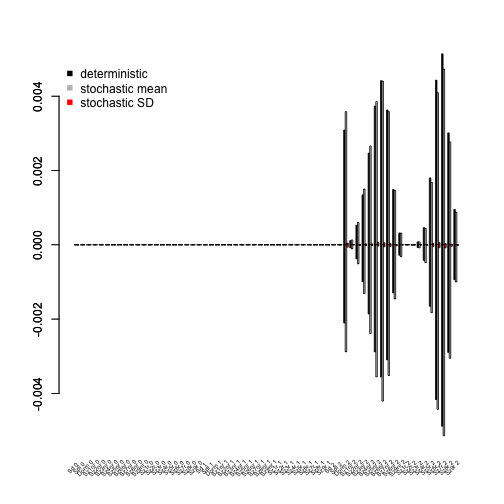Figure 8.12. LTRE contributions by age-stage The output above shows that flowering adults in the 7th size class exert the strongest influence on both $$\lambda$$ and $$\text{log} \lambda$$, with the latter influence being through the impact of shifts in the mean. The 6th flowering adult stage, and 6th non-flowering adult stage, were also strongly influential. The overall influence of each stage seems strongly split between seemingly symmetrical positive and negative contributions, reinforcing the importance of different sorts of transitions involving the most influential stages. Finally, we will assess what transition types exert the greatest impact on population growth rate. lathmat2m_ltre_summary <- summary(lathmat2m_ltre) lathmat2m_sltre_summary <- summary(lathmat2m_sltre) ltresums_tpos <- cbind(lathmat2m_ltre_summary$ahist_det$matrix1_pos, lathmat2m_sltre_summary$ahist_mean$matrix1_pos, lathmat2m_sltre_summary$ahist_sd$matrix1_pos) ltresums_tneg <- cbind(lathmat2m_ltre_summary$ahist_det$matrix1_neg, lathmat2m_sltre_summary$ahist_mean$matrix1_neg, lathmat2m_sltre_summary$ahist_sd$matrix1_neg) barplot(t(ltresums_tpos), beside = T, col = c("black", "grey", "red"), ylim = c(-0.03, 0.03)) barplot(t(ltresums_tneg), beside = T, col = c("black", "grey", "red"), add = TRUE) abline(0, 0, lty = 3) text(cex=0.85, y = -0.04, x = seq(from = 2, to = 3.98*length(lathmat2m_ltre_summary$ahist_det$category), by = 4), lathmat2m_ltre_summary$ahist_det\$category, xpd=TRUE, srt=45)
legend("bottomleft", c("deterministic", "stochastic mean", "stochastic SD"),
col = c("black", "grey", "red"), pch = 15, bty = "n")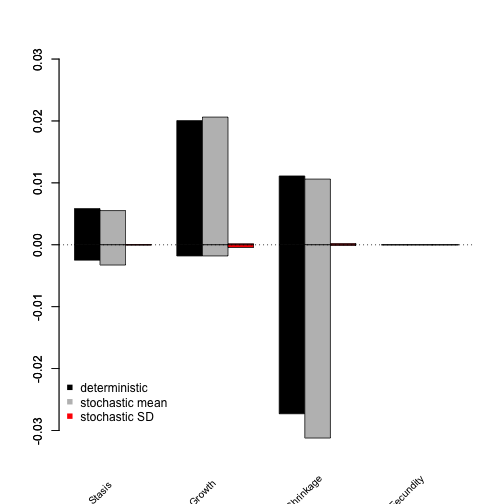Figure 8.13. LTRE contributions by transition type

The overall greatest impact on the population growth rate, whether deterministic or stochastic, appears to be from shrinkage transitions. On the whole, shifts in these transitions affected population growth rate negatively. In contrast, growth transitions exerted strong positive influence on both kinds of population growth rate, while the impacts of stasis and fecundity with small.

LTREs and sLTREs are powerful tools, and more complex versions of both analyses exist. Please consult Caswell (2001) and Davison et al. (2013) for more information.

Further analytical tools are being planned for lefko3, but packages that handle projection matrices can typically handle the individual matrices produced and saved in lefkoMat objects in this package. Differences, obscure results, and errors sometimes arise when packages are not made to handle large and/or sparse matrices - historical matrices are both, and so care must be taken with their analysis.

## Acknowledgements

We are grateful to two anonymous reviewers whose scrutiny improved the quality of this vignette. The project resulting in this package and this tutorial was funded by Grant-In-Aid 19H03298 from the Japan Society for the Promotion of Science.

## Literature cited

Bartoń, Kamil A. 2014. MuMIn: Multi-Model Inference.” https://CRAN.R-project.org/package=MuMIn.
Bates, Douglas, Martin Maechler, Ben Bolker, and Steve Walker. 2015. “Fitting Linear Mixed-Effects Models Using Lme4.” Journal of Statistical Software 67 (1): 1–48. https://doi.org/10.18637/jss.v067.i01.
Broek, Jan van den. 1995. “A Score Test for Zero Inflation in a Poisson Distribution.” Biometrics 51 (2): 738–43. https://doi.org/10.2307/2532959.
Brooks, Mollie E., Kasper Kristensen, Koen J. van Benthem, Arni Magnusson, Casper W. Berg, Anders Nielsen, Hans J. Skaug, Martin Mächler, and Benjamin M. Bolker. 2017. glmmTMB Balances Speed and Flexibility Among Packages for Zero-Inflated Generalized Linear Mixed Modeling.” The R Journal 9 (2): 378–400. https://doi.org/10.32614/RJ-2017-066.
Caswell, Hal. 2001. Matrix Population Models: Construction, Analysis, and Interpretation. Second edition. Sunderland, Massachusetts, USA: Sinauer Associates, Inc.
Davison, Raziel, Florence Nicolé, Hans Jacquemyn, and Shripad Tuljapurkar. 2013. “Contributions of Covariance: Decomposing the Components of Stochastic Population Growth in Cypripedium Calceolus.” The American Naturalist 181 (3): 410–20. https://doi.org/10.1086/669155.
Ehrlén, Johan. 1995. “Demography of the Perennial Herb Lathyrus Vernus. I. Herbivory and Individual Performance.” Journal of Ecology 83 (2): 287–95. https://doi.org/10.2307/2261567.
———. 2000. “The Dynamics of Plant Populations: Does the History of Individuals Matter?” Ecology 81 (6): 1675–84. https://doi.org/10.1890/0012-9658(2000)081[1675:TDOPPD]2.0.CO;2.
———. 2002. “Assessing the Lifetime Consequences of Plant-Animal Interactions for the Perennial Herb Lathyrus Vernus (Fabaceae).” Perspectives in Plant Ecology, Evolution and Systematics 5 (3): 145–63. https://doi.org/10.1078/1433-8319-00031.
Ehrlén, Johan, and Ove Eriksson. 1996. “Seedling Recruitment in the Perennial Herb Lathyrus Vernus.” Flora 191 (4): 377–83. https://doi.org/10.1016/S0367-2530(17)30744-2.
Ehrlén, Johan, and Kari Lehtilä. 2002. “How Perennal Are Perennial Plants?” Oikos 98: 308–22. https://doi.org/10.1034/j.1600-0706.2002.980212.x.
Ehrlén, Johan, and Zuzana Münzbergová. 2009. “Timing of Flowering: Opposed Selection on Different Fitness Components and Trait Covariation.” The American Naturalist 173 (6): 819–30. https://doi.org/10.1086/598492.
Ehrln, Johan, and Jan Van Groenendael. 2001. “Storage and the Delayed Costs of Reproduction in the Understorey Perennial Lathyrus Vernus.” Journal of Ecology 89 (2): 237–46. https://doi.org/10.1046/j.1365-2745.2001.00546.x.